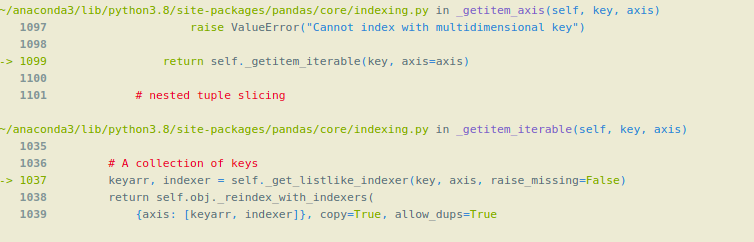GFG App
Open AppBrowser
Continue

# How to use Hierarchical Indexes with Pandas ?

The index is like an address, that’s how any data point across the data frame or series can be accessed. Rows and columns both have indexes, rows indices are called index and for columns, it’s general column names.

### Hierarchical Indexes

Hierarchical Indexes are also known as multi-indexing is setting more than one column name as the index. In this article, we are going to use homelessness.csv file.

## Python3

 `# importing pandas library as alias pd  ` `import` `pandas as pd ` ` `  `# calling the pandas read_csv() function. ` `# and storing the result in DataFrame df ` `df ``=` `pd.read_csv(``'homelessness.csv'``) ` ` `  `print``(df.head())`

Output:In the following data frame, there is no indexing.

Columns in the Dataframe:

## Python3

 `# using the pandas columns attribute. ` `col ``=` `df.columns ` `print``(col)`

Output:

Index([‘Unnamed: 0’, ‘region’, ‘state’, ‘individuals’, ‘family_members’,
‘state_pop’],
dtype=’object’)

To make the column an index, we use the Set_index() function of pandas. If we want to make one column an index, we can simply pass the name of the column as a string in set_index(). If we want to do multi-indexing or Hierarchical Indexing, we pass the list of column names in the set_index().

Below Code demonstrates Hierarchical Indexing in pandas:

## Python3

 `# using the pandas set_index() function. ` `df_ind3 ``=` `df.set_index([``'region'``, ``'state'``, ``'individuals'``]) ` ` `  `# we can sort the data by using sort_index() ` `df_ind3.sort_index() ` ` `  `print``(df_ind3.head(``10``)) `

Output:Now the dataframe is using Hierarchical Indexing or multi-indexing.

Note that here we have made 3 columns as an index (‘region’, ‘state’, ‘individuals’ ). The first index ‘region’ is called level(0) index, which is on top of the Hierarchy of indexes, next index ‘state’ is level(1) index which is below the main or level(0) index, and so on. So, the Hierarchy of indexes is formed that’s why this is called Hierarchical indexing.

We may sometimes need to make a column as an index, or we want to convert an index column into the normal column, so there is a pandas reset_index(inplace = True) function, which makes the index column the normal column.

Selecting Data in a Hierarchical Index or using the Hierarchical Indexing:

For selecting the data from the dataframe using the .loc() method we have to pass the name of the indexes in a list.

## Python3

 `# selecting the 'Pacific' and 'Mountain'  ` `# region from the dataframe. ` ` `  `# selecting data using level(0) index or main index. ` `df_ind3_region ``=` `df_ind3.loc[[``'Pacific'``, ``'Mountain'``]] ` ` `  `print``(df_ind3_region.head(``10``)) `

Output:We cannot use only level(1) index for getting data from the dataframe, if we do so it will give an error. We can only use level (1) index or the inner indexes with the level(0) or main index with the help list of tuples.

## Python3

 `# using the inner index 'state' for getting data. ` `df_ind3_state ``=` `df_ind3.loc[[``'Alaska'``, ``'California'``, ``'Idaho'``]] ` ` `  `print``(df_ind3_state.head(``10``)) `

Output:Using inner levels indexes with the help of a list of tuples:

Syntax:

`df.loc[[ ( level( 0 )  ,  level( 1 )  , level( 2 )  )  ]]`

## Python3

 `# selecting data by passing all levels index. ` `df_ind3_region_state ``=` `df_ind3.loc[[(``"Pacific"``, ``"Alaska"``, ``1434``), ` `                                    ``(``"Pacific"``, ``"Hawaii"``, ``4131``), ` `                                    ``(``"Mountain"``, ``"Arizona"``, ``7259``), ` `                                    ``(``"Mountain"``, ``"Idaho"``, ``1297``)]] ` `df_ind3_region_state `

Output:My Personal Notes arrow_drop_up